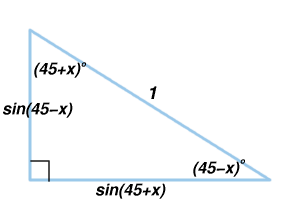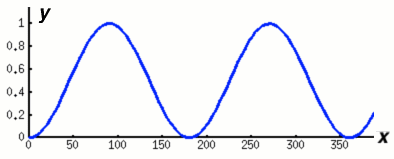#### You may also like### Telescoping Series

Find $S_r = 1^r + 2^r + 3^r + ... + n^r$ where r is any fixed positive integer in terms of $S_1, S_2, ... S_{r-1}$.### OK! Now Prove It

Make a conjecture about the sum of the squares of the odd positive integers. Can you prove it?### Overarch 2

Bricks are 20cm long and 10cm high. How high could an arch be built without mortar on a flat horizontal surface, to overhang by 1 metre? How big an overhang is it possible to make like this?

# Degree Ceremony

##### Age 16 to 18 Challenge Level:In this diagram the right angled triangle has hypotenuse of length 1 unit so the lengths of the sides are $\sin(45-x)^o$ and $\sin(45+x)^o$. By Pythagoras Theorem: $$\sin^2(45-x)^o + \sin^2(45+x)^o = 1.$$ These pairings of values that add up to 1 are useful in evaluating the expression: $$A = \sin^2 1^o + \sin^2 2^o + ... + \sin^2 359^o + \sin^2 360^\circ.$$

Ella Ryan from Madras College, St Andrew's based her solution on the symmetries of the graph of $y=\sin^2 x$.Consider the graph of $y=\sin^2 x^o$ between $x=1$ and $x=89$ inclusive and pairs of points having $y$ values which, when added together, always equal one. This result is equivalent to Pythagoras Theorem as explained above. For example, $$\sin^2 50^o + \sin^2 40^o = 1.$$ The pairs of points can be labelled $(45-x)^o$ and $(45+x)^o$ or alternatively $x^o$ and $(90-x)^o$.

Essentially the same method was used both by Ella and also by Hou Yang Yang, Millfield School, Somerset, U.K.

Firstly we use the following symmetry properties of the sine function: $$\sin^2 1^o = \sin^2 179^0 = \sin^2 181^o = \sin^2 359^o,$$ $$\sin^2 2^o = \sin^2 178^o = \sin^2 182^o = \sin^2 358^o, ...$$ $$\sin^2 89^o = \sin^2 91^o = \sin^2 269^o =\sin^2 271^o$$ and also $\sin^2 90^o = \sin^2 270^o = 1$ and $\sin 180^o = \sin 360^o = 0.$ Pairing equal values in $A$ gives:

\begin{eqnarray} A &=& 2(\sin^2 1^o + \sin^2 2^o + ... + \sin^2 179^o),\\ &=& 4(\sin^2 1^o + \sin^2 2^o + ... + \sin^2 89^o) + 2\sin^2 90^o . \end{eqnarray}

Then taking pairs that add up to 1 we get:
\begin{eqnarray} A &=& 4[(\sin^2 1^o + \sin^2 89^o) + (\sin^2 2^o + \sin^2 88^o) + ... \\ && + (\sin^2 44^o + \sin^2 46^o) + \sin^2 45^o] + 2\\ &=& 4[44 + 0.5] + 2 \\ &=& 180. \end{eqnarray}